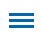With the introduction of volume, fifth-grade students learn to measure three-dimensional space. Recognizing volume and understanding it as a measurement of a space involving cubic units is an important skill. Wixie includes a Swimming Pool Volume template to provide opportunities for a guess-and-check calculation of volume in a real world context.

Editor's Note: This blog is part of a series of posts from former math specialist Scott Loomis on the real world math templates he has created for Wixie and how you can use and modify them for your own awesome performance tasks. You can read the backstory on his experience and expertise below.

## Swimming Pool Volume

The Swimming Pool Volume template outlines volume as a measurement for determining the size/space of swimming pools. After identifying the formula for volume, students are required to calculate the area of a given rectangular prism pool. In fact, all pool designs in this template are for pools in the shape of rectangular prisms. After calculating volume for a given pool, students create additional pool designs -- identifying the length, width, and height.

It may be helpful to complete the first two pages as a class so you can confirm that students understand the task of designing pools that require the exact amount of water (480 cubic feet) to fill them. Modify the template with additional pool designs if you want students to practice additional volume calculations.

Instructional Concept: Calculating Volume

Location in Templates Library: Curriculum > Math > Measurement

Estimated Completion Time: 10-20 minutes

## Math Standards and Learning Goals

### Common Core Standards - Grade 5 Mathematics - Measurement and Data

5.MD.C.5.B - Apply the formulas V = l × w × h and V = b × h for rectangular prisms to find volumes of right rectangular prisms with whole-number edge lengths in the context of solving real world and mathematical problems.

### Virginia Standards of Learning - Mathematics - Measurement and Geometry

5.8 The student will a) solve practical problems that involve perimeter, area, and volume in standard units of measure

### Texas Essential Knowledge and Skills (TEKS) Geometry and measurement

5.6 The student applies mathematical process standards to understand, recognize, and quantify volume. The student is expected to:

(B) determine the volume of a rectangular prism with whole number side lengths in problems related to the number of layers times the number of unit cubes in the area of the base.

## Wixie Skills Review

Page 2 involves the use of the Pencil tool to 'write' the formula for calculating the volume of a rectangular prism. If preferred, this can also be done by using the Text button to insert a text object.

Page 3-5 requires students to resize a rectangular prism to reflect the length, width, and height of an appropriate representation of a pool design that equals 480 cubic feet. To do this, students will need to select (single click) the object before moving the light blue arrows in the desired direction.Students will then add the length of each side with a pencil or text object. Although students may opt to eliminate the object and draw a design free-hand, the image provides an efficient option to generate applicable pool designs/models.

Page 5 provides the option of adding additional pages to construct additional designs. To make copies of any page, move to the page, go to the Edit menu and choose Duplicate.

Topics: Wixie, math, real world of math